Huang, Z., Chen, Z., Huang, D., Chui, Y., and Bian, Y. (2018). "An inelastic model for analyzing intermediately slender engineered bamboo/wood columns subjected to biaxial bending and compression," BioRes. 13(2), 2814-2833.

#### Abstract

The engineered bamboo/wood composites (EBWCs) studied in this work included solid wood, wood-based composites, and bamboo-based composites. The basic characteristic of these products is that they have similar stress-strain relationships in the parallel to the grain direction because of their similar microscopic structures. The asymmetric stress-strain relationship in tension and compression presents a great challenge for the inelastic analysis of intermediately slender EBWC columns. In this study, a novel model was developed for the inelastic analysis of biaxially loaded intermediately slender EBWC columns with rectangular cross sections. The model provides a step by step method to evaluate the nonlinear responses and load-carrying capacities of these columns. Experiments on parallel strand bamboo columns loaded with biaxial eccentric loads were conducted to validate the model. Good agreement between the experimental and predicted results was achieved. The innovative elements of the model were the asymmetric properties of EBWCs in tension and compression, and its simplicity, which lends itself to implementation in engineering design calculations. The present work is an extension of a previous study by Huang et al. (2015a), and its objective was to develop an innovative inelastic analysis model evaluating biaxially loaded intermediately slender EBWC columns with rectangular cross sections.

An Inelastic Model for Analyzing Intermediately Slender Engineered Bamboo/Wood Columns Subjected to Biaxial Bending and Compression

Zirui Huang,a,b Zhongfan Chen,a,* Dongsheng Huang,c,d Ying Hei Chui,e and Yuling Bian f

The engineered bamboo/wood composites (EBWCs) studied in this work included solid wood, wood-based composites, and bamboo-based composites. The basic characteristic of these products is that they have similar stress-strain relationships in the parallel to the grain direction because of their similar microscopic structures. The asymmetric stress-strain relationship in tension and compression presents a great challenge for the inelastic analysis of intermediately slender EBWC columns. In this study, a novel model was developed for the inelastic analysis of biaxially loaded intermediately slender EBWC columns with rectangular cross sections. The model provides a step by step method to evaluate the nonlinear responses and load-carrying capacities of these columns. Experiments on parallel strand bamboo columns loaded with biaxial eccentric loads were conducted to validate the model. Good agreement between the experimental and predicted results was achieved. The innovative elements of the model were the asymmetric properties of EBWCs in tension and compression, and its simplicity, which lends itself to implementation in engineering design calculations. The present work is an extension of a previous study by Huang et al. (2015a), and its objective was to develop an innovative inelastic analysis model evaluating biaxially loaded intermediately slender EBWC columns with rectangular cross sections.

Keywords: Biaxial bending; Beam-columns; Inelastic analysis; Engineered bamboo; Wood composite

Contact information: a: Key Laboratory of RC & PC structures of Ministry of Education, Southeast University, 02, Sipailou, Nanjing, 210096, P.R. China; b: School of Civil Engineering, Southeast University, 2, Sipailou. Nanjing, 210096, P.R. China; c: School of Civil Engineering, Nanjing Forestry University, 159, Longpan Rd, Nanjing, 210037, P.R. China; d: Jiangsu Co-Innovative Center for Efficient Application of Forestry Resource, 159, Longpan Rd, Nanjing, 210037, P.R. China; e: Department of Civil & Environmental Engineering, University of Alberta, Edmonton, T6G1H9, Canada; f: Wuxi Institute of Commerce, 809, Qianhu Rd., Wuxi, 214153, P.R. China; *Corresponding author: 101003944@seu.edu.cn

INTRODUCTION

The essential characteristic of intermediately slender columns is that the load-carrying capacity is usually dominated by the strength of the material and slenderness of the column. Numerous studies have been implemented to develop models for evaluating the in-plane load-carrying capacities of intermediately slender columns (Chen and Atsuta 1977; Buchanan 1984; Song and Lam 2009; Theiler et al. 2013; Huang et al. 2015a) since the pioneering work of von Karman on plastic performances of beam-columns (Timoshenko 1953). The plastic behaviors of biaxially loaded beam-columns have been studied extensively since the 1960s. Because of the expanding needs in the design of steel and concrete structures, many of these research studies were valuable in the U.S. and coordinated by the U.S. Column Research Council (Chen and Atsuta 1977). However, the methods developed were based on the determination of the exact deflection shape of the columns, which limited their practical use. By establishing force equilibrium conditions only at the mid-height of the column, assuming the deflection shape of the column is a half sine wave, and idealizing the material as elastic-perfectly plastic, many researchers have created approximate models to evaluate the ultimate load-carrying capacities for steel and concrete columns (Chen and Atsuta 1977). Nevertheless, these models are not applicable for bamboo and wood columns because of the complicated constitutive law of these materials. Many timber design standards use empirical compression-moment interaction equations to evaluate beam-columns (EN 1995-1-1 2004; CSA 086-14 2014; ANSI/AWC 2015).

For engineered bamboo/wood composites (EBWCs), including solid woods, wood-based composites, and bamboo-based composites, the constitutive law of the materials usually contains more complicated characteristics than for homogenous materials, especially for asymmetric properties under tension and compression (Buchanan 1984; Huang et al. 2015b, 2016; Li et al. 2016). It was reported by Buchanan (1984) that the nonlinear stress-strain relationship in the compression of solid wood was determined as early as 1840. Since then many nonlinear models have been proposed to simulate the stress-strain relationship of wood in the parallel to the grain direction (Buchanan 1984). The bilinear model is the simplest constitutive law among them, which states that wood has a bilinear elastic-plastic stress-strain relationship in compression, whereas it is elastic in tension (Neely 1898). Zakić (1973) proposed a parabolic model for solid wood, which assumed that the compressive stress-strain relationship may be approximated by parabolic curves.

Wood-based composites, such as laminated veneer lumber, laminated strand lumber, and parallel strand lumber, have been increasingly used as construction materials in the last few decades because of concerns about saving forestry resources (U.S. Department of Agriculture 2010). These composites are manufactured by bonding wood strands or fibers together with an adhesive. They usually provide more uniform and predictable in-service performances than solid wood products because of smaller and more evenly distributed flaws compared to a member of solid wood. In addition, they may provide more predictable in-service behavior because of dispersion and the elimination of defects during the manufacturing process. Although the mechanical properties of most wood-based composites might be changed compared with those of solid wood, the stress-strain relationship of solid wood is still the same as for wood-based composites (Li et al. 2016), i.e., linearity in tension and nonlinearity in compression.

In recent years, bamboo-based composites have been commercially produced and are starting to be used as construction materials in China because of the restriction on forest harvesting. Parallel strand bamboo (PSB) (Huang et al. 2015b) and laminated bamboo (Huang et al. 2016) are the major bamboo-based composites commonly used in building construction. Similar to wood-based composites, bamboo-based composites are usually fabricated by gluing bamboo strands, fiber bundles, or bamboo panels together under a controlled temperature and pressure. It has been confirmed by Huang et al.(2015b) and Huang et al. (2016) that the parallel to the grain stress-strain relationships of bamboo-based composites also present linearity in tension and nonlinearity in compression.

Indeed, bamboo and wood have similar microstructures, which consist of long and parallel cellulosic fibers embedded in ligneous tissues (Zhou et al. 2012; CSA 086-14 2014). This is photographically illustrated in Fig. 1. It is the reason why the constitutive relationships of solid woods, wood-based composites, and bamboo-based composites exhibit similarities in the parallel to the grain direction. Therefore, from the macro-mechanical perspective, solid wood, wood-based composites, and bamboo-based composites can be thought to belong to the same material category. The parallel to the grain stress-strain relationship of EBWCs may be simulated by a unique model with different values for the model parameters for different composites. Consequently, the analysis of beam-columns made of solid wood, wood-based composites, and bamboo-based composites may be performed with the same model.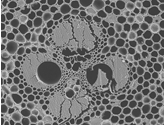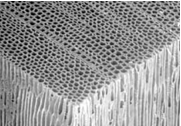(a)                                            (b)

Fig. 1. Similar microscopic structures of wood and bamboo: (a) typical microscopic structure of soft wood (U.S. Department of Agriculture 2010); and (b) microscopic structure of Phyllostachys, a common bamboo species used to manufacture bamboo-based composites

Newlin (1940) suggested a parabolic interaction equation as a failure criterion for wood columns subjected to combined in-plane bending and compression. This interaction curve is actually empirical in nature because it was based on the data fitting of experimental results. A strain-based model that takes the nonlinearities and P-delta effect into consideration for the inelastic analysis of glulam columns was proposed by Blaß (1986). The model assumes the strain over the failure section is known, and hence the stress distribution over the failure section can be inferred in accordance with the stress-strain relationship. Theiler et al. (2013) proposed a formula for the load-carrying capacity calculation of eccentrically loaded timber columns. To determine the load-carrying capacity and ultimate deflection of intermediately slender columns of bamboo-based composites, Huang et al. (2015a) developed an inelastic model to evaluate the in-plane performances of PSB columns subjected to bending and compression, in which compressive nonlinearity of the stress-strain relationship was modeled by a parabolic curve. The ultimate load-carrying capacity and deflection can be obtained with the model, and furthermore, the nonlinear responses can be traced via a step by step calculation.

More recently, studies have been done to evaluate the nonlinear performances of biaxially loaded beam-columns. Most of them postulated an elastic-perfectly plastic stress-strain relationship for materials to mitigate mathematical difficulties (Pallarés et al. 2009; Baptista 2012; Fossenti and Papia 2012; Papanikolaou 2012). Song and Lam (2009) reported a nonlinear model to evaluate the stability of timber beam-columns subjected to biaxial eccentric compression. The compressive nonlinearity of the stress-strain relationship was modeled by a 3rd order polynomial, and a numerical integration approach was employed to solve the problem.

The present work is an extension of a previous study by Huang et al. (2015a), and its objective was to develop an innovative inelastic analysis model evaluating biaxially loaded intermediately slender EBWC columns with rectangular cross sections. Solid wood, wood-based composites, and bamboo-based composites were uniquely treated as EBWCs because of their similar constitutive laws in the parallel to the grain direction. By taking the nonlinear behavior of the material into consideration, a nonlinear model was developed for determining the ultimate load-carrying capacity of intermediately slender EBWC columns loaded with a biaxial eccentric force. A key innovative element of the model was that the asymmetric properties of the EBWCs in tension and compression were considered, and it did not involve complicated mathematical calculations. Thus, the model can be easily adopted for design calculations. The model was validated by experiments on PSB columns with an eccentric load applied in a biaxial manner.

THEORETICAL MODEL

Stress-strain Relationship

The analytical model developed in the present work was based on Euler’s beam theory; hence, only the mechanical properties in the longitudinal direction, i.e., parallel to the grain, were considered. The stress-strain relationship of the solid wood in the parallel to the grain direction has been extensively studied for decades. Four constitutive models are commonly used to account for the nonlinearity of compression parallel to the grain, which are schematically illustrated in Fig. 2. The bilinear elastic-plastic and bilinear-softening stress-strain relationships proposed by Neely (1898) and Malhotra and Bazan (1980), respectively, are popular among the four models. These two models consider the proportional limit to be the maximum compressive strength; hence, they may remarkably underestimate the load-carrying capacity of members. Zakić (1973) observed the nonlinear hardening process of compression and proposed a parabolic model for the compressive stress-strain relationship of solid wood. It has been reported that the researcher Glos further took the softening process of compression into consideration and proposed a polynomial stress-strain relationship (Buchanan 1984).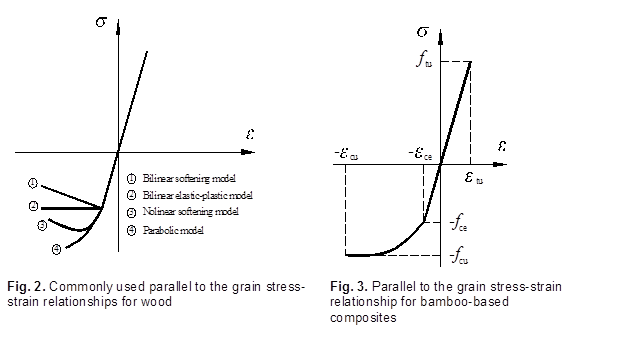Usually the parabolic and polynomial models can provide a good approximation for solid wood in compression parallel to the grain. The present study employed a parabolic model created in the study by Huang et al. (2015b) to account for the parallel to the grain stress-strain relationship of EBWCs. The equation of the stress-strain relationship may be expressed as: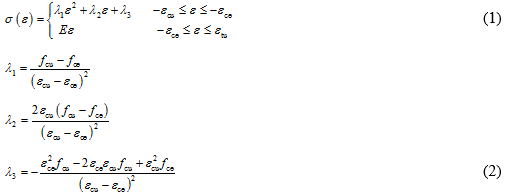where λi (i = 1, 2, and 3) is the material constant, fcu and εcu are the ultimate compressive strength (kN) and associated strain, respectively; fce and εce are the stress (MPa) and strain corresponding to the proportional compressive limit, respectively; ftu and εtu are the ultimate tensile strength (kN) and strain, respectively; and E is the Young’s modulus parallel to the grain (MPa).

The stress-strain curve is schematically shown in Fig. 3.

Analysis of the Failure Cross Section

A rectangular cross section of a column loaded with an axial force was adopted to build the governing equations. The eccentricities exand ey (Fig. 4a) were considered the Cartesian coordinates in the plane of the failure cross section, which originated from the centroid of the cross section and took the x– and y-axis to be the edges parallel to the section. Further, the load condition that is shown in Fig. 4a can be equivalent to the condition that is presented in Fig. 4b, in which the section is subjected to a centroid axial load (N) combined with biaxial moments (Mx and My), with respect to the x– and y-axis. The tensile force (N) and bending moments (Mx and My) follow the right-hand cork screw and are considered to be positive in the longitudinal direction.

The following assumptions were applied in the development of the analytical methods: (1) the plane section remains plane after deformation, (2) damage begins at the outermost corner of the compressive zone until the fiber is broken at the remote corner of the tensile zone, and (3) the neutral axis of the cross section keeps its original direction during the damage process. Thus, the strain at any point (xy) over the cross section can be functionally expressed as,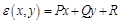(3)

where PQ, and R are the parameters that depend on the stress state of the cross section.

Furthermore, the equation of the neutral axis of the biaxial bending cross section can be expressed as: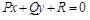(4)

Obviously, the neutral axis is a straight line. Hereafter, functional expressions for the axial compression were created with respect to the material properties, dimensions of the cross section, and eccentricities of the thrust in the linear and nonlinear states.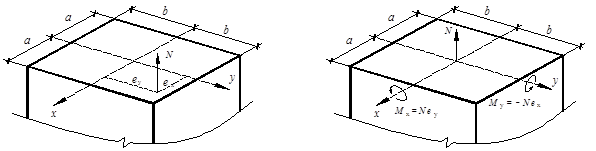(a)                                                                (b)

Fig. 4. Biaxially loaded cross section: (a) rectangular cross section subjected to a biaxial eccentric force (N); and (b) equivalent loading condition of (a)

Elastic analysis

In the elastic condition, the stress-strain relationship over the whole cross section satisfies Hooke’s law. Thus, the stress over the cross section may be expressed as: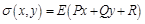(5)

The resultant forces of the cross section satisfy the equations: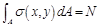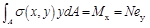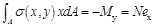(6)

Substituting Eq. 5 into Eq. 6 yields: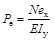,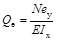, and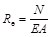(7)

where A is the area of the cross section (mm2), and Ix and Iy are the moments of inertia (mm4) about the x– and y-axis, respectively. The PQ, and R parameters of the strain plane in the elastic state were replaced by PeQe, and Re, respectively, in Eq. 7 for the sake of distinguishing the plane parameters in the elastic-plastic state, which will be discussed later. It was deduced from the plane hypothesis that the fiber at the corner of the compressive zone of the cross section (Fig. 4) must be the first one that reaches the proportional limit. After that, the cross section is turned to an elastic-plastic state. Therefore, the proportional limit of a biaxial compressive load may be computed by: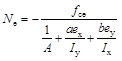(8)

Nonlinear analysis

It was deduced from the plane hypothesis that stress at the point farthest from the neutral axis should reach the yielding value first. For EBWCs, the tensile strength is usually noticeably higher than the proportional limit of compression; hence, the damage must begin from the remote corner of the compressive zone. Considering this damage mechanism and the stress-strain relationship, it was inferred that the failure cross section might be divided into three zones during the damage process, i.e., the elastic tensile zone (ETZ), elastic compressive zone (ECZ), and plastic compressive zone (PCZ), which are illustrated in Fig. 5. Obviously, the strains in the boundary of the ECZ and PCZ must equal the strain of the proportional limit of compression. It was therefore easy to deduce that the boundary of the ECZ and PCZ must be a straight line and parallel to the neutral axis, otherwise the plane hypothesis would be violated. Obviously, there were two possible PCZ shapes, triangular and trapezoidal, depending on the ratio of ex to ey. In this study, the equations based on the triangular PCZ case were derived first, and then the equations for the trapezoidal PCZ case were derived.

Assuming the boundary of the ECZ and PCZ go through points (a0b) and (ab0) for a triangular PCZ (Fig. 6a), the strain plane of a damaged cross section must satisfy the following conditions,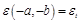, and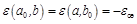(9)

where εt is the tensile strain at the remote corner of the tensile zone.

By substituting the three terms from Eq. 9 into Eq. 4, the equations to determine PQ, and for the strain plane can be obtained. Simultaneously solving the equations and considering Hooke’s law in the ETZ and ECZ yields: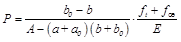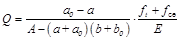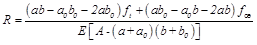(10)

Thus, the plane of strain can be determined by the intersection points of the ECZ-PCZ boundary and cross section edges. In contrast, if the distances from points (-a, –b) and (a0b) or (ab0) to the neutral axis are denoted as d1 and d2, respectively, then ft/fce must equal d1/d2according to the plane hypothesis. Thus, the relationship of the tensile stress (ft) at point (-a, –b) in the tensile zone and the compressive stress (fce) at point (a0b) in the compressive zone can be expressed as: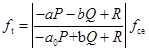(11)

The axial resultant force (N) of the cross section can be computed by,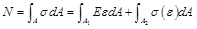(12)

where A1 and A2 denote the areas (mm2) of the elastic response zone and plastic response zone in the cross section, respectively, as is illustrated in Fig. 6.

The integration of the last term on the right side of Eq. 12 would be incredibly complicated to perform if the nonlinear stress-strain relationship was not directly substituted into the equation. Hence, a simplified approach from the previous study by Huang et al. (2013) was employed to calculate the stress integration over the PCZ. In this approach, a homogeneous coefficient (α) was introduced to consider the uneven distribution of compressive stress in the PCZ. Thus,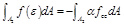was obtained. It was easy to prove that the α coefficient has the same value as that used for in-plane beam-columns (Appendix A). Therefore, α may be calculated by (Huang et al. 2013):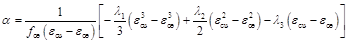(13)

where the λi coefficients are determined by Eq. 2.

Thus, Eq. 12 can be further expressed as: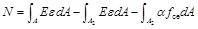(14)

In Eq. 14, an equivalent transformation for the integration over A1was conducted for the sake of reducing the difficulty of integration over an irregular area, i.e.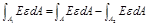. It was assumed that the equation of the ECZ-PCZ boundary has the form,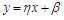(15)

where: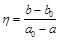,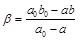(16)

After substituting the parameters obtained via Eq. 10 into Eq. 14 and considering the integral limit specified by Eq. 15, the resultant force over the cross section was obtained: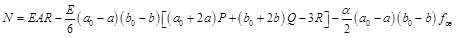(17)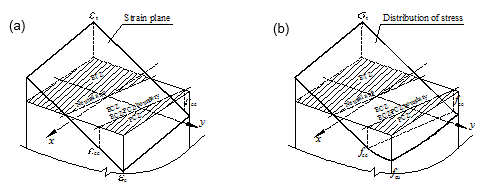Fig. 5. Typical strain (a) and stress (b) diagrams of a biaxially loaded rectangular cross section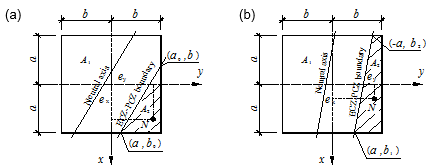Fig. 6. Critical cross sections with different shapes for the PCZ: (a) triangular PCZ; and (b) trapezoidal PCZ

The derivation of Eq. 17 can be seen in Appendix B. Considering the parallel condition of the neutral axis and ECZ-PCZ boundary, the relation of the quantities of the two intersection points a0 and b0 can be expressed as: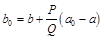(18)

According to the assumption that the neutral axis always keeps its original direction during the damage process, the following relation was obtained: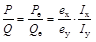(19)

Thus: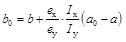(20)

It was concluded from Eqs. 10, 17, and 20 that the axial force can be determined if the intersection points of the boundary and edges of the cross section are determined. The intersection quantity a0 can be determined by the equilibrium conditions of the axial force and moments over the cross section. However, the complicated integral operations and solving of high order equations make it unlikely in practice. Indeed, the damage process of the column began with the fiber yielding at the remote corner of the PCZ and ended the moment the fibers at the remote corner of the ETZ failed. In other words, a0began at a and ended at the value corresponding to ft equals ftu at the remote corner of the ETZ. Thus, the nonlinear responses of the column can be traced step by step and the ultimate load-carrying capacity (Nu) can consequently be determined with the condition that ft equals ftu. The step by step calculation method is detailed in the Calculation Procedure section.

For the critical cross section with a trapezoidal PCZ (Fig. 6b), the parameters of the strain plane can be determined by: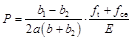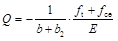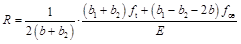(21)

where b1 and b2 are the quantities of the intersection points of the boundary and edges in the cross section, respectively.

Again, considering the parallel condition for the neutral axis yields: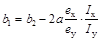(22)

Substituting Eq. 21 into Eq. 14 and considering the trapezoidal area presented in Fig. 6b for A2 yields the resultant force of the cross section of a trapezoidal PCZ, which may be expressed as: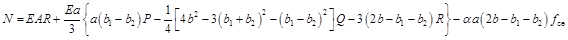(23)

The above analysis indicated that the neutral axis of bending must be a line and its direction is determined by the dimensions of the cross section and the eccentricities of compression, as is given by Eq. 19. The boundary line between the ECZ and PCZ is always parallel to the neutral axis during the damage process. If the intersections of the boundary and cross-sectional edges, i.e.a0 and b0 in Eq. 17 and a1and b1 in Eq. 23, are determined, the ultimate compressive load may be computed by Eq. 17 for a triangular PCZ and by Eq. 23 for a trapezoidal PCZ. The calculation procedure is discussed in the Calculation Procedure section.

Lateral Deflection

A pinned-end column of a certain length (l) loaded with a biaxial compressive load with eccentricities e0x and e0y was considered (Fig. 7). Theoretically, the plastic deflection can be determined through the moment-curvature equation. However, it is difficult to do in practice because the plastic curvature not only depends on the material and shape of the cross section, but also on the damage process of the cross section. Indeed, damage of a column loaded with only eccentric compression takes place in the vicinity of the failure cross section. In other words, the moment-curvature equations of elastic beam-columns (Eq. 24) still hold true the majority of the column.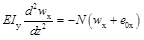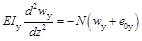(24)

where wx and wy are the deflections (mm) with respect to the x– and y-axis, respectively.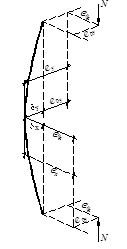Fig. 7. Deformation of a biaxially loaded column

Based on this, Huang et al. (2015a) proposed a model that considers a beam-column to be an elastic rod with a plastic hinge at the critical cross section. The total deflection of the column may be expressed as,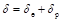(25)

where δe and δp are the fictitious elastic deflection (mm) and plastic deflection (mm), respectively.

The fictitious elastic deflection may be calculated by the elastic beam theory (Bažant and Cedolin 1991). It has been confirmed that the fictitious plastic deflection is so trivial that it can be neglected (Huang et al. 2015a). Thus, the deflections of a biaxially loaded beam-column in this study can be approximately evaluated by,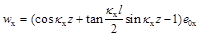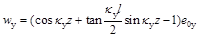(26)

where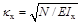and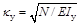. Consequently, the eccentricities of the axial force at the failure cross section at the mid-height of the column can be evaluated by substituting z with l/2 in Eq. 26 and the initial eccentricities, which may be expressed as: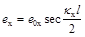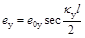(27)

Calculation Procedure

As was discussed in the Elastic Analysis section above, to determine the boundary between the ECZ and PCZ by the equilibrium conditions is inappropriate in practice because of the difficulty of solving high order equations. In fact, the damage of the cross section begins with the fiber yielding at the remote corner of the PCZ. While keeping a straight line that is always parallel to the neutral axis, the responses of this boundary originate from the remote corner of the PCZ and move toward the inside of the section during the damage process. Because the direction of the separatrix is determined by the neutral axis, the position of the separatrix can be uniquely determined by one of the intersection points, such as (a0b) for a triangular PCZ and (ab1) for a trapezoidal PCZ. Furthermore, the axial force must have a one-to-one correspondence with the position quantity (aor b1) for certain eccentricities. Thus, Eqs. 17 and 23 provide a step by step method for the nonlinear analysis of biaxially loaded beam-columns.

For the sake of simplicity and without losing generality, the following discussions were based on the case of a triangular PCZ. For a trapezoidal PCZ, the calculation procedure was the same as that for the triangular PCZ case, provided that Eqs. 10, 17, and 20 are replaced by Eqs. 21, 23, and 22, respectively.

Following the above discussions, it seemed logical that the nonlinear responses of the column can be smoothly traced step by step via Eq. 17 because the parameters of the corresponding strain plane (PiQi, and Ri) and tensile stress (fti) can be calculated via Eqs. 10 and 11 for any given a0i, and consequently Ni can be obtained through Eq. 17. However, a vicious circular problem arose because the PiQi, and Riparameters are determined by fti, and the determination of the fticontrarily relied on the PiQi, and Ri parameters. This problem can be solved by a numerical approach, such as the stationary iteration method. Nevertheless, numerical iteration is usually tedious in practice and sometimes may not achieve convergence depending on the choice for the initial value.

To determine the tensile stress (fti) for a given a0i is a critical problem during calculation. The value of the fti depends on the distance from the remote corner of the ETZ to the neutral axis of the cross section. It has been confirmed that the neutral axis is continuously offset from its original position towards the convex side during the damage process (Huang et al. 2013). However, the amount of offsetting is small; hence, PiQi, and Ri may be computed by replacing fti with fti-1 in Eq. 10 without any noticeable error, provided that the increment (Δa0i = a0i – a0-i) is small enough. Thus, a step by step procedure to trace the nonlinear responses and determine the load-carrying capacity of a biaxially loaded beam-column can be developed in this way. The calculation procedure is summarized below.

Step 1 is to determine the proportional limit of the axial force (NeviaEq. 8. In this step, the additional eccentricities caused by the elastic deformation are small and can be neglected; hence, the initial eccentricities e0x and e0y are adopted for the initial calculation.

Step 2 is to compute P0Q0, and R0 via Eq. 7 for the initial calculation. The Δa0 should be small enough that the error induced by replacing fti with fti-1 is negligible.

For step 3, a0i equals a – ii equals 1, 2, etc., fti is computed (Eq. 11), and then PiQi, and Ri are computed via Eq. 10.

Step 4 determines the boundary of the PCZ and ECZ. First, κxi and κyi are computed, and then the eccentricities exi and eyi are computed (Eq. 27). Consequently, the other intersection point (b0i) can be determined by Eq. 20.

Step 5 determines the axial load (Ni) for each step via Eq. 17.

Step 6 returns to step 3 for the next cycle of calculations. The steps are repeated until ft equals ftu.

The axial load corresponding to the last cycle should be the ultimate load-carrying capacity of the column. The flow chart for the calculation procedure is presented in Fig. 8.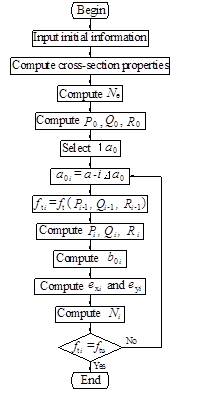Fig. 8. Flow chart of the calculation procedure

EXPERIMENTAL VALIDATION

To validate the model developed above, two groups of PSB columns subjected to biaxial eccentric compressive loads were tested. Parallel strand bamboo is manufactured by gluing parallel bamboo strands together under a controlled temperature and pressure. As was discussed above, PSB composites exhibit the typical properties of EBWCs and are well suited for use as beams and columns in frame structures for buildings. All of the experimental samples had the same square cross section of 100 mm × 100 mm, and different lengths were tested (Table 2). The dimensions of the test columns were determined in such a way that the slenderness ratios of the columns fell into the range of intermediate slenderness. Huang et al. (2015a) shows how to determine the slenderness ratio of PSB columns.

The parallel to the grain tension and compression properties of the PSB composites were tested according to the standard test method ASTM D 143-14 (2014).

RESULTS AND DISCUSSION

Table 1 presents the test results of the 12 specimens for each group of tension and compression tests.

The test columns were pin supported through two steel boots at the two ends. A biaxial eccentric compressive load induced from the actuator of a test machine was applied to the upper end, as is illustrated in Fig. 9a.

Table 1. Parallel to the Grain Properties of the PSB Composites

SD – standard deviation; CV – coefficient of variation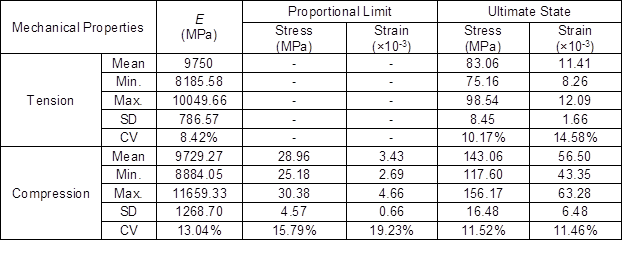A ball bearing was inserted between the plates of the boots and loading panels, which is shown in Fig. 9b. By adjusting the position of the ball bearing, any objective value for the eccentricities could be achieved.

The loading force was monotonically increased by controlling the actuator at a speed of 1 mm/min until the column collapsed. The loading force of the actuator was recorded through a TDS-530 acquisition instrument (Tokyo Sokki Kenkyujo Co., Ltd, Tokyo, Japan) at a frequency of 2 Hz. Figure 10 presents the typical failure mode of the column. It was observed that the failure was characterized by the fibers being broken at the remote corner of the tensile zone. The results of the tests and calculations are compared in Table 2. Good agreement between the measured and predicted strengths was observed.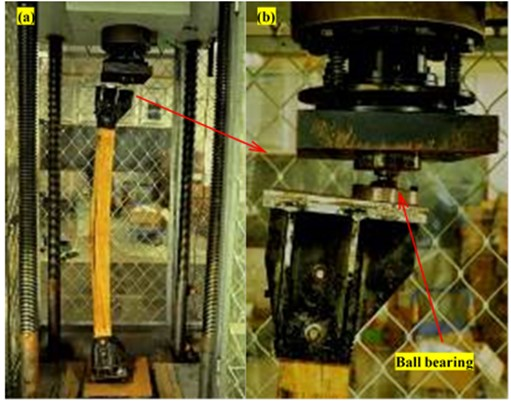Fig. 9. Test setup: (a) column loaded with biaxial compression; and (b) ball bearing, which allows for eccentricities to be adjusted to any objective value

Table 2. Comparison of the Ultimate Load-carrying Capacities of the Test Samples from the Calculations and Tests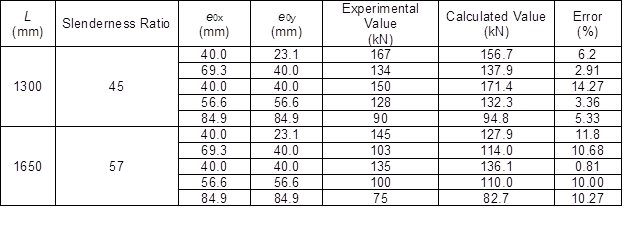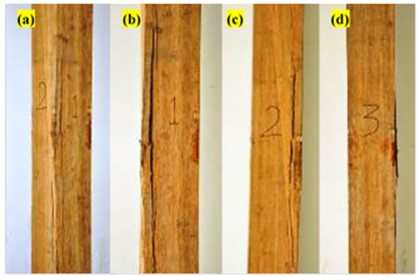Fig. 10. Typical failure mode: (a) shows the break at the remote corner of the tensile zone; (b), (c), and (d) show the front view of the failure mode of surfaces 1, 2, and 3, respectively

CONCLUSIONS

1. Solid wood, wood-based composites, and bamboo-based composites all have similar mechanical properties in a macroscopic sense because of their similar foam-fibrous microstructures. The constitutive law in the parallel to the grain direction of these materials exhibits identical characteristics to that of wood materials, i.e., linearity in tension and nonlinearity in compression; hence, they can be uniquely treated as EBWCs from a macroscopic viewpoint. The nonlinearity in compression can be approximated well with parabolic curves.
2. The load-carrying capacity of intermediately slender EBWC columns subjected to a biaxial eccentric compressive load is usually determined by the material properties and dimensions of the columns. Damage usually begins with the fibers yielding at the remote corner of the compressive zone and ends when the fibers at the remote corner of the tensile zone break.
3. Based on elementary beam theory, a novel model was developed for nonlinear analysis of intermediately slender EBWC columns with rectangular cross sections. The model provides a step by step method to trace the nonlinear responses of biaxially loaded EBWC columns. The breakthrough of this model was that the asymmetric properties of EBWCs in tension and compression were considered, and its calculation was relatively simple.
4. The accuracy of the model was validated through experimental investigation on PSB columns loaded with eccentric compressive loads. Good consistency was achieved between the results of the experiments and calculations. This implied that the effects of torsion and warping are negligible for biaxially loaded intermediately slender columns with rectangular cross sections.

ACKNOWLEDGMENTS

This research was supported by the National Science Fund of China (No. 51578291) and the Priority Academic Program Development of Jiangsu Higher Education Institutions.

REFERENCES CITED

ANSI/AWC (2015). National Design Specification for Wood Construction, American Wood Council, Leesburg, VA.

ASTM D143-14 (2014). “Standard test methods for small clear specimens of timber,” ASTM International, West Conshohocken, PA.

Baptista, A. M. (2012). “Analytical evaluation of the elastic and plastic resistances of double symmetric rectangular hollow sections under axial force and biaxial bending,” Int. J. Non-Linear Mech.47(9), 1033-1044. DOI: 10.1016/j.ijnonlinmec.2012.06.008

Bažant, Z. P., and Cedolin, L. (1991). Stability of Structures: Elastic, Inelastic, Fracture, and Damage Theories, Oxford University Press, New York, NY.

BlaßH. J. (1986). “Strength model for glulam columns,” in: International Council for Research and Innovation in Building and Construction, Vancouver, Canada.

BlaßH. J. (1987). “Design of timber columns,” in: Internal Council for Research and Innovation in Building and Construction, Vancouver, Canada.

Buchanan, A. H. (1984). Strength Model and Design Method for Bending and Axial Load Interaction in Timber Members, Ph.D. Thesis, University of British Columbia, Vancouver, Canada.

Chen, W.-F., and Atsuta, T. (1977). Theory of Beam-columns Volume 2: Space Behavior and Design, McGraw-Hill, Inc., New York, NY.

EN 1995-1-1 (2004). “Eurocode 5: Design of timber structures – Part 1-1: General – Common rules and rules for buildings,” European Committee for Standardization, Brussels, Belgium.

Fossenti, M., and Papia, M. (2012). “Dimensionless analysis of RC rectangular sections under axial load and biaxial bending,” Eng. Struct. 44, 34-45. DOI: 10.1016/j.engstruct.2012.05.049

Huang, D., Bian, Y., Huang, D. M., Zhou, A., and Sheng, B. (2015a). “An ultimate-state-based-model for inelastic analysis of intermediate slenderness PSB columns under eccentrically compressive load,” Constr. Build. Mater. 94, 306-314. DOI: 10.1016/j.conbuildmat.2015.06.059

Huang, D., Bian, Y., Zhou, A., and Sheng, B. (2015b). “Experimental study on stress-strain relationships and failure mechanisms of parallel strand bamboo made from phyllostachys,” Constr. Build. Mater. 77, 130-138. DOI: 10.1016/j.conbuildmat.2014.12.012

Huang, D., Zhou, A., and Bian, Y. (2013). “Experimental and analytical study on the nonlinear bending of parallel strand bamboo beams,” Constr. Build. Mater. 44, 585-582. DOI: 10.1016/j.conbuildmat.2013.03.050

Huang, Z., Chen, Z., Huang, D., and Zhou, A. (2016). “The ultimate load-carrying capacity and deformation of laminated bamboo hollow decks: Experimental investigation and inelastic analysis,” Constr. Build. Mater. 117, 190-197. DOI: 10.1016/j.conbuildmat.2016.04.115

Li, Z., He, M., Tao, D., and Li, M. (2016). “Experimental buckling performance of scrimber composite columns under axial compression,” Compos. Part B-Eng. 86, 203-213. DOI: 10.1016/j.compositesb.2015.10.023

Malhotra, S. K., and Bazan, I. M. M. (1980). “Ultimate bending strength theory for timber beams,” Wood Sci. 13(1), 50-63.

Neely, S. T. (1898). “Relation of compression-endwise to breaking load of beam,” in: Progress in Timber Physics, USDA Forest Service, Washington, D.C., pp. 13-17.

Newlin, J. A. (1940). “Formulas for columns with side loads and eccentricity,” Building Standards Monthly.

Pallarés, L., Miguel, P. F., and Fernández-Prada, M. A. (2009). “A numerical method to design reinforced concrete sections subjected to axial forces and biaxial bending based on ultimate strain limits,” Eng. Struct. 31(12), 3065-3071. DOI: 10.1016/j.engstruct.2009.08.006

Papanikolaou, V. K. (2012). “Analysis of arbitrary composite sections in biaxial bending and axial load,” Comput. Struct. 98-99, 33-54. DOI: 10.1016/j.compstruc.2012.02.004

Papanikolaou, V. K., and Sextos, A. G. (2016). “Design charts for rectangular R/C columns under biaxial bending: A historical review toward a Eurocode-2 compliant update,” Eng. Struct. 115, 196-206. DOI: 10.1016/j.engstruct.2016.02.033

Song, X., and Lam, F. (2009). “Laterally braced wood beam-columns subjected to biaxial eccentric loading,” Comput. Struct. 87(17-18), 1058-1066. DOI: 10.1016/j.compstruc.2009.04.007

Theiler, M., Frangi, A., and Steiger, R. (2013). “Strain-based calculation model for centrically and eccentrically loaded timber columns,” Eng. Struct. 56, 1103-1116. DOI: 10.1016/j.engstruct.2013.06.032

Timoshenko, S. (1953). History of Strength of Materials, McGraw-Hill, Inc., New York, NY.

U.S. Department of Agriculture (2010). Wood HandbookWood as an Engineering Material (Centennial Edition), Forest Products Laboratory, Madison, WI.

Zakić, B. D. (1973). “Inelastic bending of wood beams,” J. Struct. Div.-ASCE 99(10), 2079-2095.

Zhou, A., Huang, D., Li, H., and Su, Y. (2012). “Hybrid approach to determine the mechanical parameters of fibers and matrixes of bamboo,” Constr. Build. Mater. 35, 191-196. DOI: 10.1016/j.conbuildmat.2012.03.011

Article submitted: December 3, 2017; Peer review completed: January 14, 2018; Revised version received: February 18, 2018; Accepted: February 19, 2018; Published: February 26, 2018.

DOI: 10.15376/biores.13.2.2814-2833

APPENDICES

Appendix A

The homogenous coefficient (α) for in-plane bending beam-columns is defined as: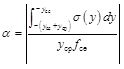(A1)

For biaxial bending beam-columns, α is defined as: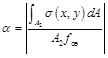(A2)

where yce and ycp are the depths (mm) of the ECZ and PCZ for the in-plane bending column, and A2 is the area (mm2) of the PCZ of the biaxial bending column. For the sake of simplicity, the integral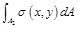was converted to the coordinates ξ – χ in the plane of the cross section, which assumes the ξ-axis is parallel to the ECZ-PCZ boundary, as is illustrated in Fig. A1. Thus, the stress at any point in the PCZ should only depend on the distance of the point to the separatrix, and can be expressed as: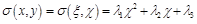(A3)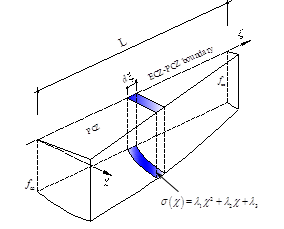Fig. A1. Coordinate transformation during the calculation of the resultant force over the PCZ

The resultant force over the PCZ can be calculated by: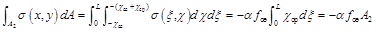(A4)

Hence, it was concluded that the homogeneous coefficients for in-plane and out-of-plane bending are the same.

Appendix B

For convenience, Eq. 17 was rewritten as: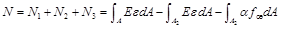(B1)

Substituting Eq. 3 for the first two terms of Eq. B1 yields: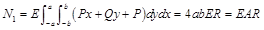(B2)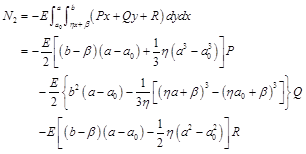(B3)

The last term of Eq. B1 may be expressed as: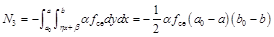(B4)

The boundary condition of the separatrix gives:

ηa0 + β = b (B5)

ηa + β = b0 (B6)

Substituting these two equations into Eq. B3 yields: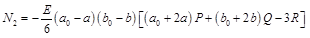(B7)

Thus Eq. 17 can be obtained by substituting Eqs. B2, B3, and B7 into Eq. B1.

For a trapezoidal PCZ, the integration of the second and third term of Eq. B1 is expressed as Eqs. B8 and B9, respectively: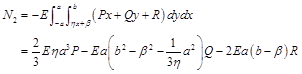(B8)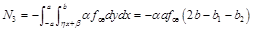(B9)

The linear conditions of the intersections of the separatrix and edges of the cross section yield the equations:

aη + β = b1 (B9)

-aη + β = b2 (B10)

Substituting these two equations into Eqs. B8 and B9, and considering that the first term of Eq. B1 is identical for both triangular and trapezoidal PCZs, the equation for calculating the resultant force in the case of a trapezoidal PCZ (Eq. 23) can be obtained.